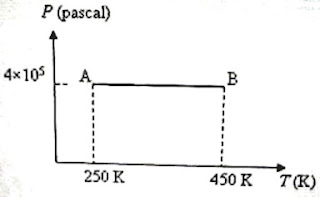## Pages

`“Life is like riding a bicycle.  To keep your balance you must keep moving.”–Albert Einstein`

## Tuesday, May 26, 2009

### AP Physics B – Multiple Choice Questions on Kinetic Theory and Thermodynamics

Everything that is really great and inspiring is created by the individual who can labour in freedom

– Albert Einstein

Equations to be remembered in kinetic theory of gases were discussed in the post dated 13th March 2008, followed by a discussion of some typical multiple choice questions (for practice) in the post dated 17th march 2008. You can access those posts by clicking on the label ‘kinetic theory’ below this post.

Important points related to thermodynamics were discussed in the post dated 21st May 2008, followed by a discussion of some typical multiple choice questions (for practice) in the post dated 26th May 2008. A couple of free response practice questions on thermodynamics were discussed in the post dated 3rd June 2008. You can access them by clicking on the label ‘thermodynamics’ below this post.

Today we will discuss a few more multiple choice questions on Kinetic Theory and Thermodynamics.

(1) A region of the earth’s atmosphere contains n molecules (treated as ideal gas molecules) per unit volume. The temperature of air in the region is T. If k represents Boltzmann’s constant and R represents universal gas constant, the pressure of air in the region is

(a) nT/k

(b) nkT

(c) RT/n

(d) nRT

(e) nRkT

Since PV = μRT where μ is the number of moles in the volume V of the gas at the pressure P and temperature T, we have

P = (μRT)/V

Since k = R/N where N is Avogadro number, R = Nk.

Therefore, P = (μNkT)/V.

But (μN)/V = n, the number of molecules per unit volume so that P = nkT

[The expression for the pressure P of a gas according to the kinetic theory is

P = (1/3) nmc2

The r.m.s.velocity c of the molecule is √(3kT/m) where m is the molecular mass. On substituting for c in the expression for P you can easily obtain the expression P = nkT].

(2) An ideal monoatomic gas is heated so that it expands at constant pressure. What percentage of the heat supplied to the gas is used to increase the internal energy of the gas?

(a) 100 %

(b) 0 %

(c) 60 %

(d) 50 %

(e) 40 %

Since a monoatomic gas molecule has 3 degrees of freedom, the molar heat capacity (also called molar specific heat) of the monoatomic gas at constant volume (CV) is (3/2)R where R is universal gas constant. Its molar heat capacity at constant pressure (CP) is (5/2)R . This follows from Meyer’s relation, CP = CV + R.

This means that when heat energy equal to (5/2)R joule is supplied to one mole of a monoatomic gas to make it expand at constant pressure, (3/2)R joule is used to increase the temperature by 1 K and the remaining R joule is used for doing work against the forces which oppose the expansion. 3/2 is 60 % of 5/2. So the correct option is (c).

(3) Oxygen and nitrogen in two enclosures have the same mass, volume and pressure. The ratio of the temperature of oxygen to that of nitrogen is

(a) 7/8

(b) 8/7

(c) 1

(d) 49/64

(e) 64/49

We have PV = μRT where μ is the number of moles in the volume V of the gas at the pressure P and temperature T.

Since P and V are the same for the two gases,

PV = μ1RT1 = μ2RT2 where μ1 and μ2 are the numbers of moles of oxygen and nitrogen and T1 and T2 are their temperatures respectively.

Therefore, μ1T1 = μ2T2 so that T1/T2 = μ2/μ1

The samples of the two gases have the same mass (say, m).

Therefore, μ1 = m/0.032 and μ2 = m/0.028 since the molar masses of oxygen and nitrogen are 0.032 kg and 0.028 kg respectively.

Therefore, T1/T2 = μ2/μ1 = 0.032/0.028 = 8/7.

(4) In a Carnot engine 7000 J of heat is absorbed from a source at 400 K and 4900 J of heat is rejected to the sink. The temperature of the sink is

(a) 200 K

(b) 220 K

(c) 260 K

(d) 280 K

(e) 340 K

We have Q1/T1 = Q2/T2 with usual notations.

Substituting the given values, 7000/400 = 4900/T2.

Therefore, T2 = (4900×400)/7000 = 280 K(5) Three moles of an ideal monoatomic gas is initially in the state A shown in the adjoining pressure-temperature graph. It is taken to state B without changing its pressure. If R is the universal gas constant, the work done by the gas in this process is

(a) 200 R

(b) 300 R

(c) 400 R

(d) 500 R

(e) 600 R

The work done by the gas in taking it from state A to state B = P∆V where ∆V is the increase in volume at constant pressure P.

We have PV = μRT where μ is the number of moles in the sample of the gas and R is the universal gas constant.

Therefore we have

P∆V = μR ∆T = 3×R(450 – 250) = 600R [Option (e)].

Find a few more multiple choice questions (with solution) on thermodynamics here.

1.Hello, your web site is very useful for High School Physics Student.

I want to ask if the answer to question 3 is wrong?(the oxygen nitrogen question)

2.